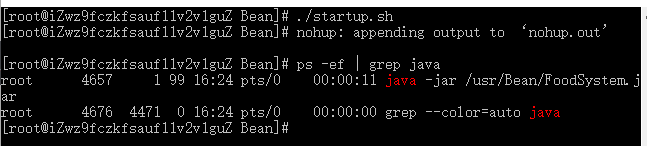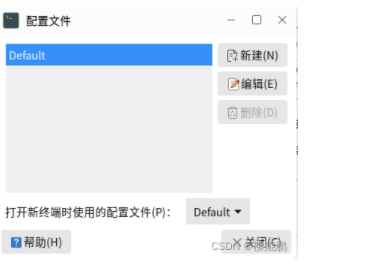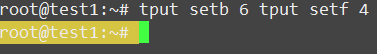+关注继续查看

# 问题发现# Linux终端颜色显示设置

\033[显示方式;前景色;背景色m

• 1
• 1

# 个性化Ubuntu Linux终端

## 设置

PS1="$\033[0;32m$\A $\033[0;31m$\u$\033[0;34m$@$\033[0;35m$\h$\033[0;34m$:$\033[00;36m$\W$\033[0;33m$ $$\033[0m$"  • 1 • 1 然后使用source ~/.bashrc让设置立即生效。 或者直接将以上代码输入终端，你会发现你的终端变样子了在Linux的环境变量中，PSI是 基本提示符，对root 是# 普通用户$

## 说明

\033 声明了转义序列的开始，然后是 [ 开始定义颜色。 后面的 0 定义了默认的字体宽度，接着的中间的数字定义字符颜色。最后面的数字定义了字符背景色。字母m是定义本身所必须的，字母m后面的字符就是你想改变的字符了。

PS1="$\033[0;32m$\A $\033[0;31m$\u$\033[0;34m$@$\033[0;35m$\h$\033[0;34m$:$\033[00;36m$\W$\033[0;33m$ $$\033[0m$"  • 1 • 1 首先是$\033[0;32m$\A，在终端中输入PS1=”[\033[0;32m]\A”,你看到了什么。\033[0;32m 设置字体前景色，即字体颜色为绿色 \A 会在终端中显示当前的时间 如果想让终端恢复初始设置，我们这时候只需要重新设置PSI的值，或者关闭当前终端，重新再打开一个就可以了，因为我们在终端直接设置PSId的值是即时生效，但是确是只对当前终端有效的。 接着是$\033[0;31m$\u，是将用户名显示成红色，u表示user。 下面的$\033[0;34m$@ ，是蓝色显示@字符信息。 后面的$\033[0;35m$\h，是用紫红色显示主机名，h表示hostname。 紧接着是: 号的设置显示成蓝色$\033[0;34m$: 然后是路径的显示设置，颜色是青色，$\033[00;36m$\W 继续下来是终端符号root 是#， 普通用户$ ，显示成黄色$\033[0;33m$ $ 最后就是我们自己输入的命令的设置了，默认吧，使用$\033[0m$ 关闭终端颜色的设置。 # 最后附上几个大神的设置信息 # 将您的终端变成带表格样式的 PS1="┌─[\d][\u@\h:\w]\n└─>"  • 1 • 2 • 1 • 2# 将您的终端变成表格样式 + 带颜色显示 PS1="$\033[0;32m$┌┼─┼─ $\033[0m\033[0;32m$\u$\033[0m$ @ $\033[0;36m$\h$\033[0m\033[0;32m$ ─┤├─ $\033[0m$\t \d$\033[0;32m$ ─┤├─ $\033[0;31m$\w$\033[0;32m$ ─┤ \n$\033[0;32m$└┼─$\033[0m\033[0;32m$\$$\033[0m\033[0;32m$─┤▶$\033[0m$"


• 1
• 2
• 3
• 1
• 2
• 3#  将您的终端变得个性十足
PS1="$\e[0;1m$┌─( $\e[31;1m$\u$\e[0;1m$ ) – ( $\e[36;1m$\w$\e[0;1m$ )\n└──┤ $\e[0m$"

• 1
• 2
• 1
• 216 0LINUX设置终端窗口显示内容的滚动缓冲行数
LINUX设置终端窗口显示内容的滚动缓冲行数
36 0LINUX编译mate-desktop/terminal-1.24.0终端
LINUX编译mate-desktop/terminal-1.24.0终端
23 0Linux的各种复制粘贴 - VIM、tmux、和终端之间的复制粘贴
Linux的各种复制粘贴 - VIM、tmux、和终端之间的复制粘贴
173 0LINUX开机，直接进入终端，如何加载硬盘
LINUX开机，直接进入终端，如何加载硬盘
38 0Linux terminal 终端快捷键
Linux terminal 终端快捷键
42 035 0Linux Command tput 终端操作
Linux Command tput 终端操作
59 0linux终端的快捷命令汇总
linux终端的快捷命令汇总
48 0

Linux 用户及权限11384

Linux 系统管理进阶8614

Linux 文本管理入门7125

LINUX 企业常用服务29847

Linux 运维自动化课程13514

Linux Shell 编程18374

Decian GNU/Linux安全合规之路